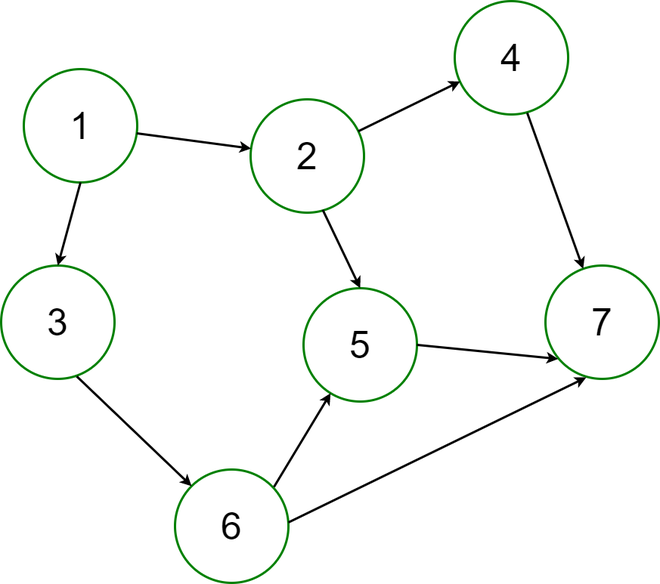Related Articles

# How to Generate a Random Directed Acyclic Graph for a Given Number of Edges in Java?

• Difficulty Level : Hard
• Last Updated : 04 Dec, 2020

A Directed Acyclic Graph is a directed graph with no directed cycles. In a directed graph, the edges are connected so that each edge only goes one way. A directed acyclic graph means that the graph is not cyclic, or that it is impossible to start at one point in the graph and traverse the entire graph. Each edge is directed from an earlier edge to a later edge.

To generate a random DAG(Directed Acyclic Graph) for a given number of edges.Directed Acyclic Graph

Examples:

```Input:
Enter the number of Edges :
20
Output:
The Generated Random Graph is :
1 -> { Isolated Vertex! }
2 -> { Isolated Vertex! }
3 -> { 18  }
4 -> { 5  }
5 -> { 16 8  }
6 -> { Isolated Vertex! }
7 -> { Isolated Vertex! }
8 -> {  }
9 -> { Isolated Vertex! }
10 -> { Isolated Vertex! }
11 -> { Isolated Vertex! }
12 -> {  }
13 -> { Isolated Vertex! }
14 -> { 18  }
15 -> { Isolated Vertex! }
16 -> {  }
17 -> { 19 3 5 4  }
18 -> {  }
19 -> {  }
20 -> { 12  }

Input:
Enter the number of Edges :
30
Output:
The Generated Random Graph is :
1 -> { 12 8 7 16 5 11  }
2 -> { 16  }
3 -> {  }
4 -> { 10  }
5 -> {  }
6 -> { 7  }
7 -> { 5  }
8 -> { 7 12 20  }
9 -> { 16 12  }
10 -> { 3  }
11 -> { 17 14  }
12 -> { 4 3  }
13 -> { 12 5  }
14 -> { 15 17  }
15 -> {  }
16 -> { 20  }
17 -> { 20 13  }
18 -> {  }
19 -> { 12 11  }
20 -> { 18  }```

Approach:

• Take the input of the number of edges for the random Directed Acyclic Graph.
• Build a connection between two random vertex and check if any cycle is generated due to this edge.
• If any cycle is found, this edge is discarded and a random vertex pair is generated again.

Implementation:

## Java

 `// Java program to Generate a Random Directed``// Acyclic Graph for a Given Number of Edges`` ` `import` `java.io.*;``import` `java.util.*;``import` `java.util.Random;`` ` `public` `class` `RandomDAG {``   ` `    ``// The maximum number of vertex for the random graph``    ``static` `int` `maxVertex = ``20``;``   ` `    ``// Function to check for cycle, upon addition of a new``    ``// edge in the graph``    ``public` `static` `boolean` `checkAcyclic(``int``[][] edge, ``int` `ed,``                                       ``boolean``[] check, ``int` `v)``    ``{``        ``int` `i;``        ``boolean` `value;``       ` `        ``// If the current vertex is visited already, then``        ``// the graph contains cycle``       ` `        ``if` `(check[v] == ``true``)``           ` `            ``return` `false``;``       ` `        ``else` `{``           ` `            ``check[v] = ``true``;``           ` `            ``// For each vertex, go for all the vertex``            ``// connected to it``            ``for` `(i = ed; i >= ``0``; i--) {``               ` `                ``if` `(edge[i][``0``] == v)``                   ` `            ``return` `checkAcyclic(edge, ed, check, edge[i][``1``]);``               ` `            ``}``        ``}``       ` `        ``// In case, if the path ends then reassign the``        ``// vertexes visited in that path to false again``        ``check[v] = ``false``;``       ` `        ``if` `(i == ``0``)``            ``return` `true``;``        ``return` `true``;``    ``}``   ` `    ``// Function to generate random graph``    ``public` `static` `void` `generateRandomGraphs(``int` `e)``    ``{``       ` `        ``int` `i = ``0``, j = ``0``, count = ``0``;``        ``int``[][] edge = ``new` `int``[e][``2``];``        ``boolean``[] check = ``new` `boolean``[``21``];``        ``Random rand = ``new` `Random();``       ` `        ``// Build a connection between two random vertex``        ``while` `(i < e) {``           ` `            ``edge[i][``0``] = rand.nextInt(maxVertex) + ``1``;``            ``edge[i][``1``] = rand.nextInt(maxVertex) + ``1``;``           ` `            ``for` `(j = ``1``; j <= ``20``; j++)``                ``check[j] = ``false``;``           ` `            ``if` `(checkAcyclic(edge, i, check, edge[i][``0``]) == ``true``)``               ` `                ``i++;``           ` `            ``// Check for cycle and if found discard this``            ``// edge and generate random vertex pair again``        ``}``       ` `        ``System.out.println(``"The Generated Random Graph is :"``);``       ` `        ``// Print the Graph``        ``for` `(i = ``0``; i < maxVertex; i++) {``           ` `            ``count = ``0``;``            ``System.out.print((i + ``1``) + ``" -> { "``);``           ` `            ``for` `(j = ``0``; j < e; j++) {``               ` `                ``if` `(edge[j][``0``] == i + ``1``) {``                    ``System.out.print(edge[j][``1``] + ``" "``);``                    ``count++;``                ``}``               ` `                ``else` `if` `(edge[j][``1``] == i + ``1``) {``                    ``count++;``                ``}``               ` `                ``else` `if` `(j == e - ``1` `&& count == ``0``)``                    ``System.out.print(``"Isolated Vertex!"``);``            ``}``           ` `            ``System.out.print(``" }\n"``);``        ``}``    ``}``   ` `    ``public` `static` `void` `main(String args[]) ``throws` `Exception``    ``{``        ``int` `e = ``4``;``       ` `        ``System.out.println(``"Enter the number of Edges :"``+ e);`` ` `        ``// Function to generate a Random Directed Acyclic``        ``// Graph``        ``generateRandomGraphs(e);``    ``}``}`
Output
```Enter the number of Edges :4
The Generated Random Graph is :
1 -> { Isolated Vertex! }
2 -> { 10  }
3 -> {  }
4 -> { Isolated Vertex! }
5 -> {  }
6 -> { 11  }
7 -> { Isolated Vertex! }
8 -> { Isolated Vertex! }
9 -> { Isolated Vertex! }
10 -> { 5  }
11 -> {  }
12 -> { Isolated Vertex! }
13 -> { Isolated Vertex! }
14 -> { Isolated Vertex! }
15 -> { 3  }
16 -> { Isolated Vertex! }
17 -> { Isolated Vertex! }
18 -> { Isolated Vertex! }
19 -> { Isolated Vertex! }
20 -> { Isolated Vertex! }
```

Attention reader! Don’t stop learning now. Get hold of all the important Java Foundation and Collections concepts with the Fundamentals of Java and Java Collections Course at a student-friendly price and become industry ready. To complete your preparation from learning a language to DS Algo and many more,  please refer Complete Interview Preparation Course.

My Personal Notes arrow_drop_up